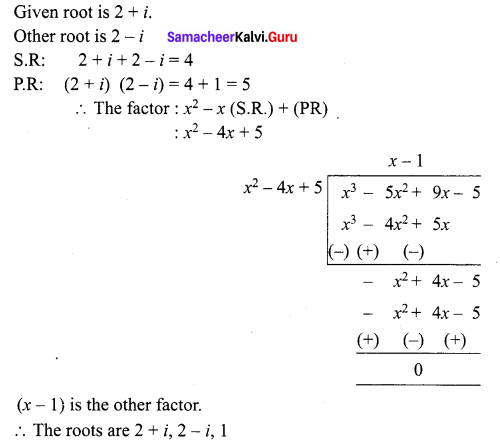# Samacheer Kalvi 12th Maths Solutions Chapter 3 Theory of Equations Ex 3.3

## Tamilnadu Samacheer Kalvi 12th Maths Solutions Chapter 3 Theory of Equations Ex 3.3

Question 1.
Solve the cubic equation: 2x3 – x2 – 18x + 9 = 0 if sum of two of its roots vanishes.
Solution:
The given equation is 2x3 – x2 – 18x + 9 = 0
$$x^{3}-\frac{x^{2}}{2}-9 x+\frac{9}{2}=0$$
Let the roots be α, -α, β
α – α + β = $$-\left(\frac{-1}{2}\right)$$
$$\Rightarrow \beta=\frac{1}{2}$$
(α) (-α) (β) = $$\frac{-9}{2}$$
$$\Rightarrow-\alpha^{2}\left(\frac{1}{2}\right)=\frac{-9}{2}$$
α2 = 9
α = ±3
The roots are 3, -3, $$\frac { 1 }{ 2 }$$

Question 2.
Solve the equation 9x3 – 36x2 + 44x – 16 = 0 if the roots form an arithmetic progression.
Solution:
The given equation is 9x3 – 36x2 + 44x – 16 = 0
$$x^{3}-4 x^{2}+\frac{44}{9} x-\frac{16}{9}=0$$
Let the roots be α – d, α, α + d
As they are in A.P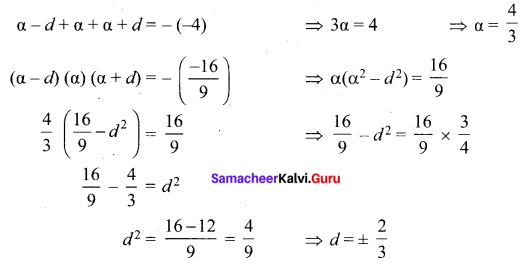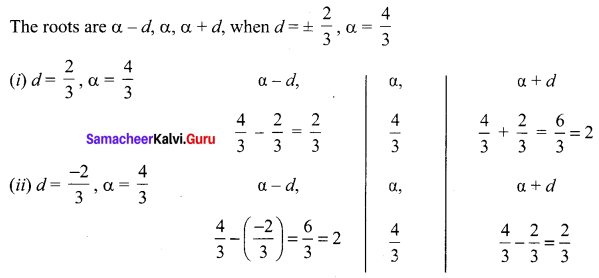Question 3.
Solve the equation 3x3 – 26x2 + 52x – 24 = 0 if its roots form a geometric progression.
Solution:
The given equation is 3x3– 26x2 + 52x – 24 = 0
$$x^{3}-\frac{26}{3} x^{2}+\frac{52}{3} x-8=0$$
Given that the root are GP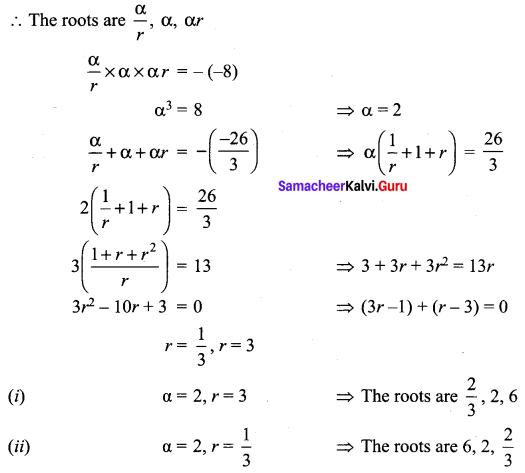Question 4.
Determine ft and solve the equation 2x3 – 6x2 + 3x + k = 0 if one of its roots is twice the sum of the other two roots.
Solution:
The given equation is 2x3 – 6x2 + 3x + k = 0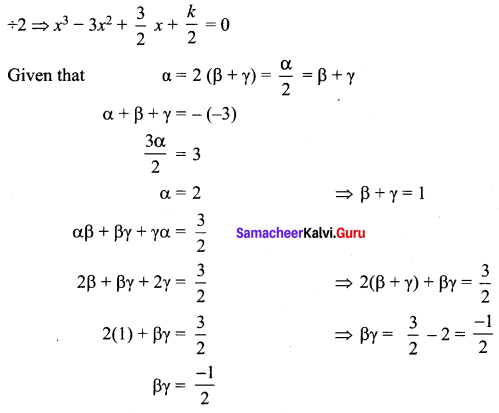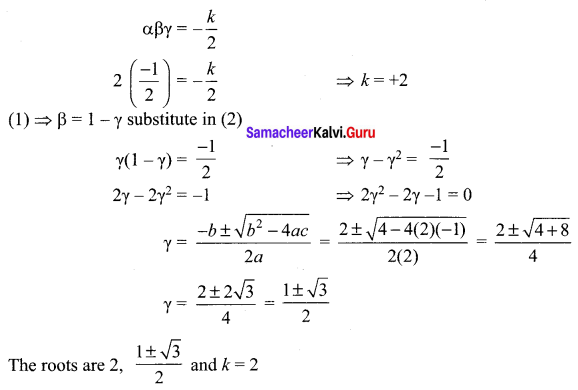Question 5.
Find all zeros of the polynomial x6 – 3x5 – 5x4 + 22x3 – 39x2 – 39x + 135, if it is known that 1 + 2i and √3 are two of its zeros.
Solution:
The given equation is x6 – 3x5 – 5x4 + 22x3 – 39x2 – 39x + 135 = 0
The given roots are 1 + 2i, √3
The other roots are 1 – 2i, -√3
The factors are
= {x2 – x(2) + (1 + 4)}{(x + √3)(x – √3)}
= (x2 – 2x + 5) (x2 – 3)
= x4 – 3x2 – 2x3 + 6x + 5x2 – 15
= x4 – 2x3 + 2x2 + 6x – 15
To find this roots,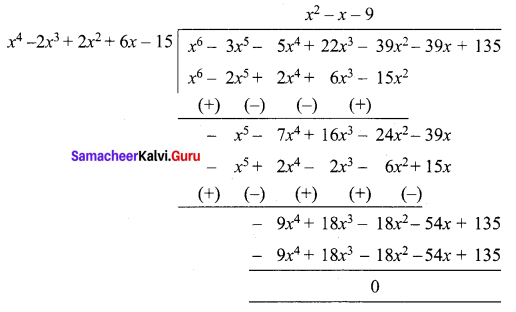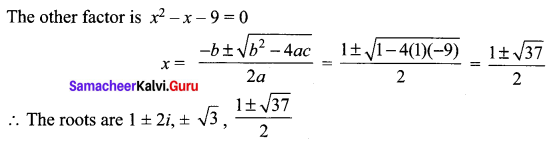Question 6.
Solve the cubic equations:
(i) 2x3 – 9x2 + 10x = 3
(ii) 8x3 – 2x2 – 7x + 3 = 0
Solution:
(i) Given equation is 2x3 – 9x2 + 10x = 3
Sum of the co-efficients = 0
(x – 1) is a factor.
The other factor is 2x2 – 7x + 3
2x2 – 7x + 3 = 0
(x – 3)(2x – 1) = 0
The roots are 1, 3, $$\frac { 1 }{ 2 }$$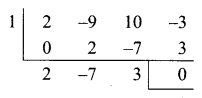(ii) Given equation is 8x3 – 2x2 – 7x + 3 = 0
Sum of odd co-efficients = Sum of even co-efficients
(x + 1) is a factor.
The other factor is 8x2 – 10x + 3
8x2 – 10x + 3 = 0
(4x – 3) (2x – 1) = 0
The roots are $$\frac{3}{4}, \frac{1}{2},-1$$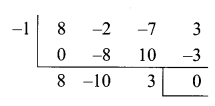Question 7.
Solve the equation: x4 – 14x2 + 45 = 0.
Solution:
The given equation is x4 – 14x2 + 45 = 0
Let x2 = y
y2 – 14y + 45 = 0
(y – 9)(y – 5) = 0
y = 9, 5
x2 = 9, x2 = 5
x = ± 3, x = ± √5
The roots are ± 3, ± √5

### Samacheer Kalvi 12th Maths Solutions Chapter 3 Theory of Equations Ex 3.3 Additional Problems

Question 1.
If one root of x3 + 2x2 + 3x + k = 0 is sum of the other two roots then find the value of k.
Solution:
Let α, β, γ be the roots of given equation.
But, α = β + γ
α + β + γ = -2 … (1) ⇒ 2α = -2 ⇒ α = -1
αβ + βγ + γα = 3 … (2) This gives β + γ = – 1
αβγ = -k …(3)Question 2.
If sum of the roots of the equation x3 – 3x2 – 16x + k = 0 is zero then find the value of k.
Solution:
Let α, β, γ be the roots of given equation.
But, α + β = 0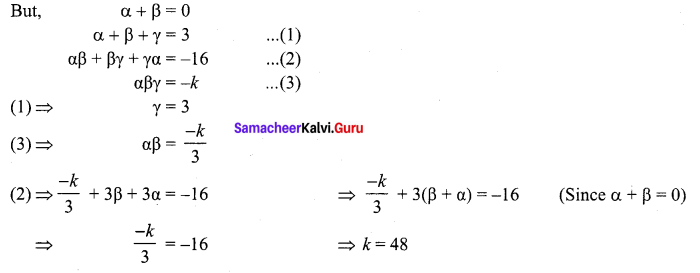Question 3.
Find all zeros of the polynomial x3 – 5x2 + 9x – 5 = 0, If 2 + i is a root.
Solution: